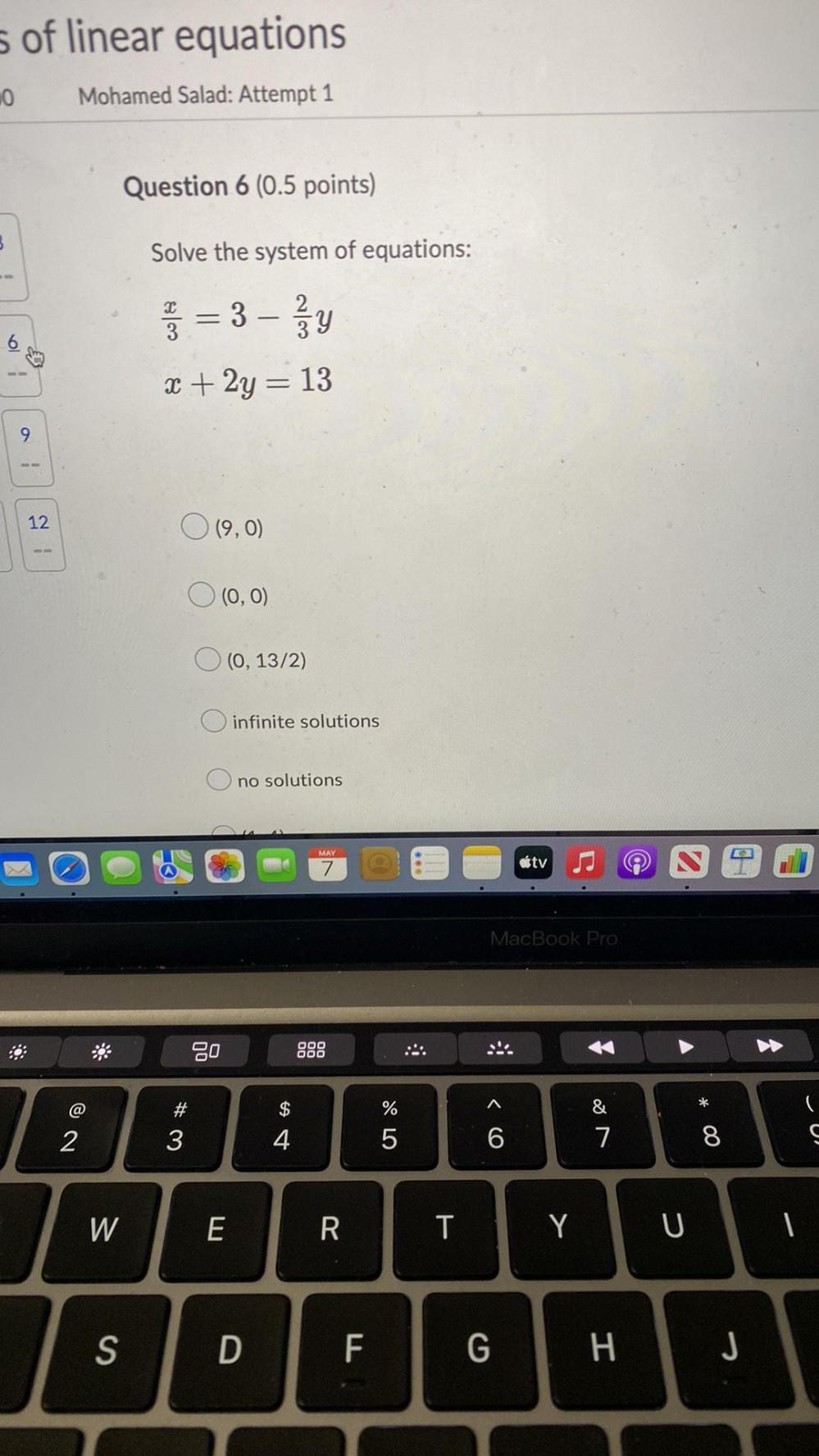Question:

# Solve the system of equations: (x/3) = 3 - (2/30 y x + 2y = 13 a. (9,0) b. (0,0) c. (0, 13/2) d. infinite solutions e. no solutionsSolve the system of equations: (x/3) = 3 - (2/30 y x + 2y = 13 a. (9,0) b. (0,0) c. (0, 13/2) d. infinite solutions e. no solutions